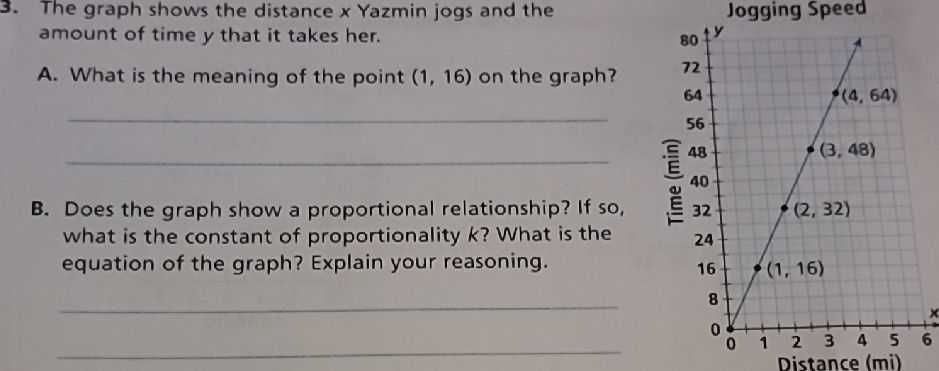### ¿Todavía tienes preguntas de matemáticas?

Pregunte a nuestros tutores expertos
Algebra
Pregunta3. The graph shows the distance $$x$$ Yazmin jogs and the amount of time $$y$$ that it takes her.

A. What is the meaning of the point $$( 1,16 )$$ on the graph?

B. Does the graph show a proportional relationship? If so, what is the constant of proportionality $$k$$ ? What is the equation of the graph? Explain your reasoning.